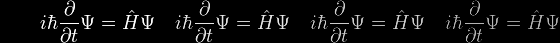# Eqn 12c Help

Given that
 y ∫ a
dy
=
 x ∫ 0
½ (ex/a – e–x/a) dx

we can represent this in terms of the antiderivatives (indefinite integrals) and their specified limits as

y
 y | a
=  (a/2) (ex/a + e–x/a)
 x | 0

which becomes

(y – a) = (a/2) {(ex/a + e–x/a) – (e0 + e0)}
(y – a) = (a/2) {(ex/a + e–x/a) – 2}

and finally

y = ½ a (ex/a + e–x/a)